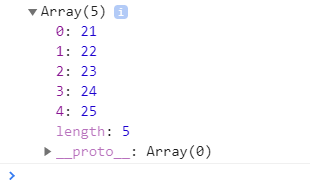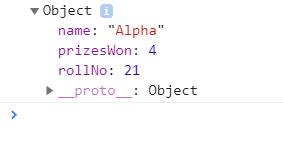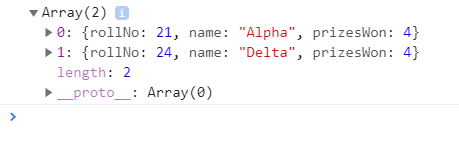GeeksforGeeks App
Open AppBrowser
Continue

# Higher-Order Arrow Functions in JavaScript

Prerequisite: Arrow Functions

A Higher-Order function is a function that receives a function as an argument otherwise returns the function as output. The higher-Order Arrow function implies using arrow functions (in ES6) along with Higher-Order functions.

Needs of Higher Order Arrow Function:

• In a general way, the programmer instructs on how to perform the function rather than what is needed which increases the length of the code and makes it error-prone.
• Whereas in the Higher Order Arrow Functions implementation, the code is much short, concise, succinct, easy to debug, and focuses on what is required rather than how to achieve it.
• We can directly work with the current value instead of accessing it individually using its index (i.e arr).
• There is no need to create a predefined array and push back the changes.
• The mutation of objects can be avoided and maintenance of for loop is not required.

Why avoid forEach()? The forEach() function does not return any value so results need to be pushed in a predefined array whereas this is not the case in the map() function.

## javascript

 `// Data set of students``var` `Students = [``    ``{ rollNo: 21, name: ``'Alpha'` `},``    ``{ rollNo: 22, name: ``'Beta'` `},``    ``{ rollNo: 23, name: ``'Gamma'` `},``    ``{ rollNo: 24, name: ``'Delta'` `},``    ``{ rollNo: 25, name: ``'Omega'``}``];`` ` `// Use forEach() function``var` `StudentRollNo = [];`` ` `Students.forEach(``function` `(Student) {``    ``StudentRollNo.push(Student.rollNo);``});`` ` `// Display rollNo data``console.log(StudentRollNo);`

Output:The Higher-Order functions are:

JavaScript map() Function: It works on a given array like changing/transforming the whole array and then simply returning it. It does not break the flow for a few conditions. The map() function takes two arguments. The first is a callback which gives the current value of the iteration, the index of the iteration, original array from which the map was called. The other argument is not mandatory which is the value to use as this in the callback. One drawback of using the map() function is that its performance is good only with small data sets

## javascript

 `// Data set of students``var` `Students = [``    ``{ rollNo: 21, name: ``'Alpha'` `},``    ``{ rollNo: 22, name: ``'Beta'` `},``    ``{ rollNo: 23, name: ``'Gamma'` `},``    ``{ rollNo: 24, name: ``'Delta'` `},``    ``{ rollNo: 25, name: ``'Omega'``}``];`` ` `// Use map() function``var` `StudentRollNo = Students.map(``function` `(Student) {``    ``return` `Student.rollNo``});`` ` `// Display rollNo data``console.log(StudentRollNo);`

1. Output:The implementation of above code using arrow functions

## javascript

 `// Data set of students``var` `Students = [``    ``{ rollNo: 21, name: ``'Alpha'` `},``    ``{ rollNo: 22, name: ``'Beta'` `},``    ``{ rollNo: 23, name: ``'Gamma'` `},``    ``{ rollNo: 24, name: ``'Delta'` `},``    ``{ rollNo: 25, name: ``'Omega'``}``];`` ` `// Use map() function with arrow functions``const StudentRollNo =``    ``Students.map(Student => Student.rollNo);`` ` `// Display Roll no data``console.log(StudentRollNo);`

Output:JavaScript reduce() Function: It is similar to the map() function in terms of a callback for every element of the array. But the difference is that it reduces passes the result of this callback from the original array to another. The result is termed as an accumulator which can be of anything integer, character, string, object, map, etc and should be passed while invoking. The callback now gets the accumulator, current value, index of iteration, and whole array. In simple words, the accumulator accumulates all the return values. Its value is the collection of previously returned accumulations.

## javascript

 `// Data set of students``var` `Students = [``    ``{ rollNo: 21, name: ``'Alpha'``, prizesWon: 1 },``    ``{ rollNo: 22, name: ``'Beta'``, prizesWon: 3 },``    ``{ rollNo: 23, name: ``'Gamma'``, prizesWon: 0 },``    ``{ rollNo: 24, name: ``'Delta'``, prizesWon: 0 },``    ``{ rollNo: 25, name: ``'Omega'``, prizesWon: 1}``];`` ` `// Using reduce() function``var` `totalPrizes = Students.reduce(``function` `(accumulator, Student) {``    ``return` `accumulator + Student.prizesWon;``}, 0);`` ` `// Display total number of prizes won by all``console.log(totalPrizes);`

Output:

`5`

The implementation of above code using arrow functions

## javascript

 `// Data set of students``var` `Students = [``    ``{ rollNo: 21, name: ``'Alpha'``, prizesWon: 1 },``    ``{ rollNo: 22, name: ``'Beta'``, prizesWon: 3 },``    ``{ rollNo: 23, name: ``'Gamma'``, prizesWon: 0 },``    ``{ rollNo: 24, name: ``'Delta'``, prizesWon: 0 },``    ``{ rollNo: 25, name: ``'Omega'``, prizesWon: 1}``];`` ` `// Using reduce() function with arrow functions``const totalPrizes = Students.reduce(``    ``(accumulator, Student) => accumulator + Student.prizesWon, 0);`` ` `// Display total number of prizes won by all``console.log(totalPrizes);`

Output:

`5`

JavaScript find() Function: It also works on an array and returns the first array element which satisfies the condition given in the function. It is similar to map() function. Its performance is not much efficient in case of large data sets although it works fine with small data sets.

## javascript

 `// Data set of students``var` `Students = [``    ``{ rollNo: 21, name: ``'Alpha'``, prizesWon: 4 },``    ``{ rollNo: 22, name: ``'Beta'``, prizesWon: 3 },``    ``{ rollNo: 23, name: ``'Gamma'``, prizesWon: 0 },``    ``{ rollNo: 24, name: ``'Delta'``, prizesWon: 4 },``    ``{ rollNo: 25, name: ``'Omega'``, prizesWon: 1}``];`` ` `// Using find() function``var` `achievers = Students.find(``function` `(Student) {``    ``return` `Student.prizesWon == 4;``});`` ` `// Display only first Student who won four prizes``console.log(achievers);`

Output:The implementation of above code using arrow functions

## javascript

 `// Data set of students``var` `Students = [``    ``{ rollNo: 21, name: ``'Alpha'``, prizesWon: 4 },``    ``{ rollNo: 22, name: ``'Beta'``, prizesWon: 3 },``    ``{ rollNo: 23, name: ``'Gamma'``, prizesWon: 0 },``    ``{ rollNo: 24, name: ``'Delta'``, prizesWon: 4 },``    ``{ rollNo: 25, name: ``'Omega'``, prizesWon: 1}``];`` ` `// Using find() function with arrow functions``var` `achievers = Students.find(``    ``(Student) => Student.prizesWon == 4);`` ` `// Display only first Student who won four prizes``console.log(achievers);`

Output:JavaScript filter() Function: The filter() function works on an array and returns an array for filtered items implying the length of the array is reduced. It also receives the similar arguments as map but the difference lies in the callback as it needs to return either true or false. If the value returned is true then the element remains in the array otherwise the element is filtered out.

## javascript

 `// Data set of students``var` `Students = [``    ``{ rollNo: 21, name: ``'Alpha'``, prizesWon: 4 },``    ``{ rollNo: 22, name: ``'Beta'``, prizesWon: 3 },``    ``{ rollNo: 23, name: ``'Gamma'``, prizesWon: 0 },``    ``{ rollNo: 24, name: ``'Delta'``, prizesWon: 4 },``    ``{ rollNo: 25, name: ``'Omega'``, prizesWon: 1}``];`` ` `// Using filter() function``var` `achievers = Students.filter(``function` `(Student) {``    ``return` `Student.prizesWon == 4;``});`` ` `// Display Students who won four prizes``console.log(achievers);`

Output:The implementation of above code using arrow functions

## javascript

 `// Data set of students``var` `Students = [``    ``{ rollNo: 21, name: ``'Alpha'``, prizesWon: 4 },``    ``{ rollNo: 22, name: ``'Beta'``, prizesWon: 3 },``    ``{ rollNo: 23, name: ``'Gamma'``, prizesWon: 0 },``    ``{ rollNo: 24, name: ``'Delta'``, prizesWon: 4 },``    ``{ rollNo: 25, name: ``'Omega'``, prizesWon: 1}``];`` ` `// Using filter() function with arrow functions``var` `achievers = Students.filter(``    ``(Student) => Student.prizesWon == 4);`` ` `// Display Students who won four prizes``console.log(achievers);`

Output:My Personal Notes arrow_drop_up
Related Tutorials